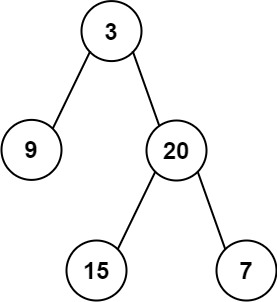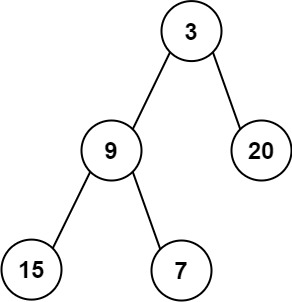### LeetCode• ㊗️
• 大家
• offer
• 多多！

## Problem

Given the root of a binary tree, return the average value of the nodes on each level in the form of an array. Answers within $10^{-5}$ of the actual answer will be accepted.

Example 1:Input: root = [3,9,20,null,null,15,7]
Output: [3.00000,14.50000,11.00000]
Explanation: The average value of nodes on level 0 is 3, on level 1 is 14.5, and on level 2 is 11.
Hence return [3, 14.5, 11].


Example 2:Input: root = [3,9,20,15,7]
Output: [3.00000,14.50000,11.00000]


Constraints:

• The number of nodes in the tree is in the range $[1, 10^4]$.
• $-2^{31} <= Node.val <= 2^{31} - 1$

## Code

class Solution {
public List<Double> averageOfLevels(TreeNode root) {
queue.offer(root);
List<Double> res = new ArrayList<>();

while(!queue.isEmpty()) {
int size = queue.size();
double sum = 0;
for(int i = 0; i < size; i++) {
TreeNode curr = queue.poll();
sum += curr.val;

if(curr.left != null) queue.offer(curr.left);
if(curr.right != null) queue.offer(curr.right);
}